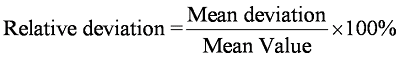## 1.4.1 Consistency, Accuracy and Sensitivity

Precision
1. Precision is the ability of an instrument in measuring a quantity in a consistent manner with only a small relative deviation between readings.
2. The precision of a reading can be indicated by its relative deviation.
3. The relative deviation is the percentage of mean deviation for a set of measurements and it is defined by the following formula:Accuracy
1. The accuracy of a measurement is the approximation of the measurement to the actual value for a certain quantity of Physics.
2. The measurement is more accurate if its number of significant figures increases.
3. Table above shows that the micrometer screw gauge is more accurate than the other measuring instruments.4. The accuracy of a measurement can be increased by

• taking a number of repeat readings to calculate the mean value of the reading.
• avoiding the end errors or zero errors.
• taking into account the zero and parallax errors.
• using more sensitive equipment such as a vernier caliper to replace a ruler.
5. The difference between precision and accuracy can be shown by the spread of shooting of a tar-get (as shown in Diagram below).Sensitivity
1. The sensitivity of an instrument is its ability to detect small changes in the quantity that is being measured.
2. Thus, a sensitive instrument can quickly detect a small change in measurement.
3. Measuring instruments that have smaller scale parts are more sensitive.
4. Sensitive instruments need not necessarily be accurate.

1. Ash Acoustic // May 14, 2011 at 7:23 PM

what is deviation?

2. Orang biasa // November 8, 2011 at 6:39 AM

what is deviation? does it really matter?

3. Grateful Ww // February 8, 2012 at 12:30 AM

Deviation is sort of "perbezaan"

4. John Liang // November 27, 2013 at 8:22 PM

deviation is a measure of the how much spread from the central point

5. Jennifer Thompson // October 20, 2019 at 7:46 AM

I’ve only tried one essay service but I can tell you that the website I used was really solid. It’s called DigitalEssay.net. Basically you get to pick a writer and you can communicate with them through an internal chat system which makes explaining how to do specific assignments a lot easier (especially if your teacher is a hard-ass like mine was.) Good luck with your paper!